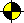The first chapter of this guide is concluded by the procedure for computing descendent of some man in the genealogy database.

descendent(D,A):-parent(A,D).
descendent(D,A):-parent(P,D),descendent(P,A).

The definition of this procedure is recursive:

• first clause for finding direct descendent concludes the recursion
• the second clause uses the call to the same procedure (i.e., recursion) to find indirect descendent.

There are more possibilities how to define the recursive clause, i.e., how to find indirect descendent. We will analyze these cases now.

Case 1

descendent(D,A):-parent(P,D),descendent(P,A).

In the above procedure we start searching with the parent P of descendent D and ask whether this parent is a descendent of the ancestor A. So, we go step by step from descendent to ancestor as the following diagram shows:

D->P->->->->->A.

This approach is advisable if one knows the descendent and looking for appropriate ancestor.

Case 2

descendent(D,A):-descendent(P,A),parent(P,D).

One can switch the order of goals in the clause. The declarative meaning of the clause remains the same but the operational behaviour changes dramatically. Now, we are looking for any descendent P of the ancestor A which is a parent of the "original" descendent D. The following diagram shows this process:

A->->->->->P->D.

This approach, when the recursive call is first, is usually not recommended because of its complexity. One have to find many descendants before the right descendent, which is a parent of D, is found.

Case 3

descendent(D,A):-parent(A,C),descendent(D,C).

It is more efficient to find descendent in ancestor to descendent order if one starts from a children of the ancestor. The following diagram shows this process:

A->C->->->->->D.

This approach is comparable with Case 1 and it is advisable if one knows the ancestor and looking for appropriate descendent. However, if we assume that the number of children is greater than 2 on the average (the number of parents is 2) then the procedure in Case 1 will be more efficient (if the descendent is known).

Case 4

descendent(D,A):-descendent(D,C),parent(A,C).

Now, one can do the same switch of goals as in Case 2. The following diagram shows the respective process:

D->->->->->C->A.

Again, this approach is not recommended and the procedure from Case 1 is much more better for search in descendent to ancestor order.

End of Recursion

In all above cases, we expect that the clause for ending recursion is the first clause in the procedure. This is usually the secure position of such clause as it guarantees that the recursion will stop.

Obversely, putting clause for ending recursion first is also less efficient as this clause is tried in each step of the recursion (by nature of Prolog execution) even if it can be used at the end of recursion only. Therefore, in some cases it is more efficient to put such clause at the end of procedure if one knows what he/she is doing. This is safe, if it is easy to distinguish, e.g., via unification, whether one should stop or continue in recursion respectively. This is usually the case of list operations as following example shows:

This procedure works fine if one is adding an element to the end of the list, i.e.,

But as soon as this procedure is used in a different way, e.g., to generate lists